# Martin Orr's Blog

## Gross's proof of the Chowla-Selberg formula

Posted by Martin Orr on Friday, 04 March 2016 at 11:40

Today I am going to write about Gross's proof of the Chowla-Selberg formula (up to algebraic numbers). As I discussed last time, the Chowla-Selberg formula is a formula for the periods of a CM elliptic curvein terms of values of the gamma function. The idea of Gross's proof is to construct a family of abelian varieties equipped with a section of the de Rham cohomology which has constant periods, such that there is one abelian variety in the family where the period is easy to calculate, and another abelian variety in the family is a power ofand so knowing a period of this variety allows us to calculate the periods of.

## Motivic Galois groups and periods

Posted by Martin Orr on Tuesday, 03 November 2015 at 16:00

In my last post, I discussed how the existence of a polarisation implies an upper bound for the transcendence degree of the extended period matrix of an abelian variety, namely the dimension of the general symplectic group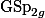(whereis the dimension of the abelian variety). In this post, I will discuss how this can be generalised to take into account all algebraic cycles on the abelian variety. The groupis replaced by the motivic Galois group of the abelian variety, which I will define. I will also mention how Deligne's theorem on absolute Hodge cycles allows us to replace the motivic Galois group by the Mumford-Tate group.

## Period relations on abelian varieties

Posted by Martin Orr on Monday, 26 October 2015 at 11:00

The Legendre period relation is a classical equation relating the periods and quasi-periods of an elliptic curve, as defined last time. I will discuss this relation, and then more generally discuss how the existence of polarisations implies relations between the periods of higher-dimensional abelian varieties.

These examples motivate the introduction of the geometric motivic Galois group, which gives an upper bound for the transcendence degree of periods of an abelian variety (or indeed any algebraic variety). This upper bound is conjectured to be equal to the actual transcendence degree. I had intended to discuss the geometric motivic Galois group in this post too, but I decided that it was getting to long so I will postpone that to another time.

## Periods of abelian varieties

Posted by Martin Orr on Tuesday, 06 October 2015 at 16:10

There are a couple of different matrices associated with an abelian variety which are referred to as its period matrix. These matrices relate different choices of bases for the tangent space orof the abelian variety. In this post I will discuss the different definitions of period matrices and how they relate to each other.

A complex abelian variety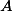can be realised as the quotient of the-dimensional complex vector spaceby the rank-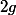lattice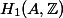. The period matrix expresses a basis of this lattice in terms of a basis for the tangent space. We can also get a period matrix which is twice as large by using de Rham cohomologyinstead of the tangent space.

The period matrix can be defined for any complex abelian variety, but it contains additional information if the abelian variety is defined over a number field, as we can then choose our basis forto also be defined over. The period matrix then gives us a set of complex numbers relating a basis of a-vector space to a basis of a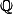-vector space, and the transcendence properties of these numbers are interesting and I will discuss them in a later post.

## Absolute Hodge classes in l-adic cohomology

Posted by Martin Orr on Friday, 26 June 2015 at 11:30

We can define absolute Hodge classes in-adic cohomology in the same way as absolute Hodge classes in de Rham cohomology. We can then prove Deligne's theorem, that Hodge classes on an abelian variety are absolute Hodge, for-adic cohomology. Because it is easy to prove that absolute Hodge classes in-adic cohomology are potentially Tate classes, this implies half of the Mumford-Tate conjecture.

In particular, it implies that ifis an abelian variety over a number field, then a finite index subgroup of the image of the-adic Galois representation onis contained in the-points of the Mumford-Tate group of. This is the goal I have been working towards for some time on this blog.

Deligne's definition of absolute Hodge classes considered-adic cohomology (for all) and de Rham cohomology simultaneously. The accounts I read of this theory focussed on the de Rham side, leading me to believe that the de Rham part was essential and the-adic part an optional extra. This is why I wrote the past few posts about de Rham cohomology and am now adding the-adic version on at the end, even though I am more interested in the-adic version. Now that I understand what is going on, I realise that I could have used only-adic cohomology from the beginning. One day I might write up a neater account which uses-adic cohomology only.

Before the main part of this post, talking about absolute Hodge classes in-adic cohomology, I need to talk about Tate twists in-adic cohomology. These are more significant than Tate twists in singular cohomology because they change the Galois representations involved. This resulted in some mistakes in my previous posts on Tate classes, which I think I have now fixed.